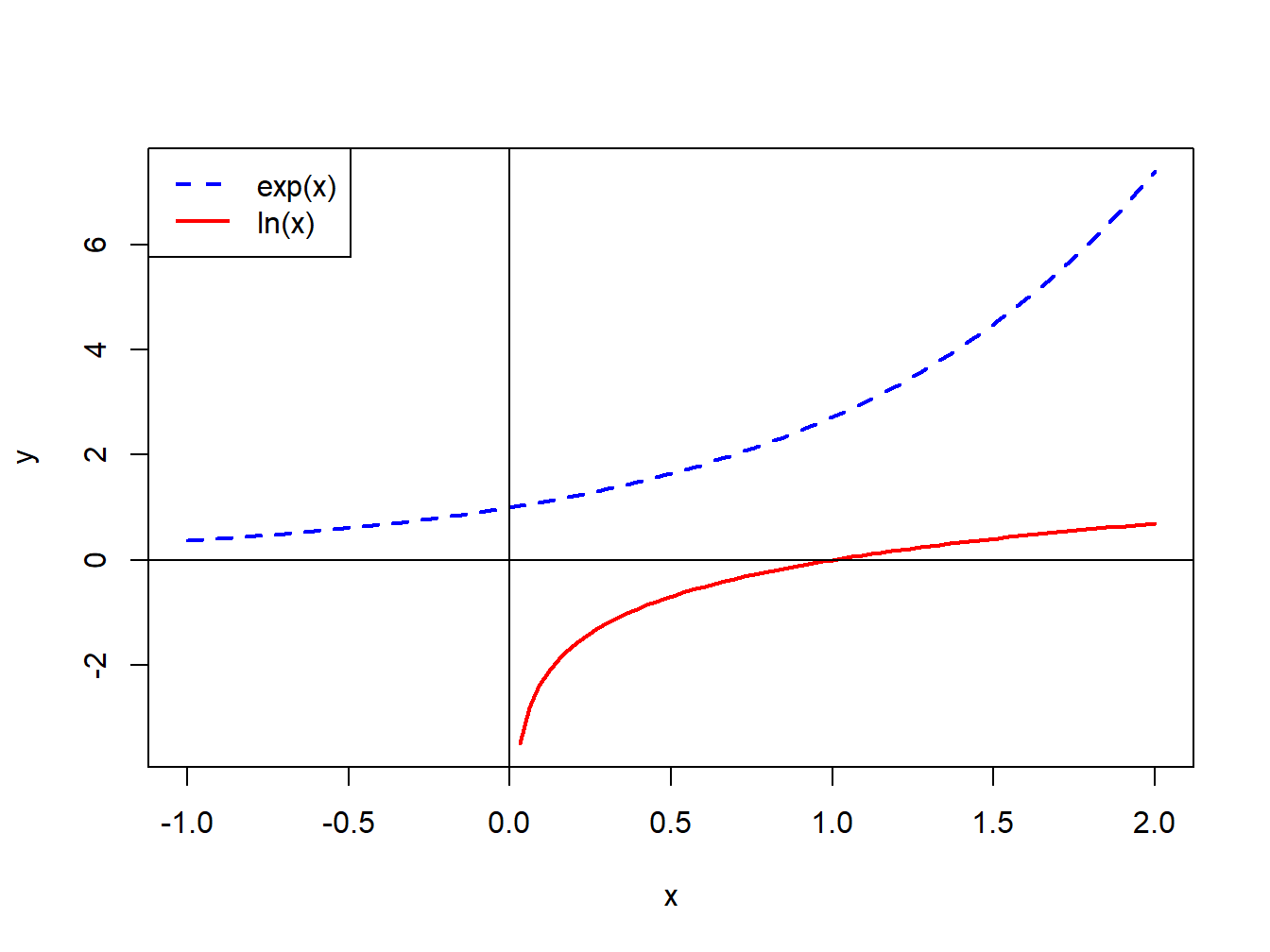## 1.6 Appendix: Properties of Exponentials and Logarithms

The computation of continuously compounded returns requires the use of natural logarithms. The natural logarithm function, $$\ln(\cdot),$$ is the inverse of the exponential function, $$e^{(\cdot)}=\exp(\cdot),$$ where $$e^{1}=2.718.$$ That is, $$\ln(x)$$ is defined such that $$x=\ln(e^{x}).$$ Figure 1.6 plots $$e^{x}$$ and $$\ln(x)$$. Notice that $$e^{x}$$ is always positive and increasing in $$x$$. $$\ln(x)$$ is monotonically increasing in $$x$$ and is only defined for $$x>0.$$ Also note that $$\ln(1)=0$$ and $$\ln(0)=-\infty.$$Figure 1.6: Exponential and natural logarithm functions.

The exponential and natural logarithm functions have the following properties:

1. $$\ln(x\cdot y)=\ln(x)+\ln(y),$$ $$x,y>0$$
2. $$\ln(x/y)=\ln(x)-\ln(y),$$ $$x,y>0$$
3. $$\ln(x^{y})=y\ln(x),$$ $$x>0$$
4. $$\frac{d\ln(x)}{dx}=\frac{1}{x},$$ $$x>0$$
5. $$\frac{d}{dx}\ln(f(x))=\frac{1}{f(x)}\frac{d}{dx}f(x)$$ (chain-rule)
6. $$e^{x}e^{y}=e^{x+y}$$
7. $$e^{x}e^{-y}=e^{x-y}$$
8. $$(e^{x})^{y}=e^{xy}$$
9. $$e^{\ln(x)}=x$$
10. $$\frac{d}{dx}e^{x}=e^{x}$$
11. $$\frac{d}{dx}e^{f(x)}=e^{f(x)}\frac{d}{dx}f(x)$$ (chain-rule)